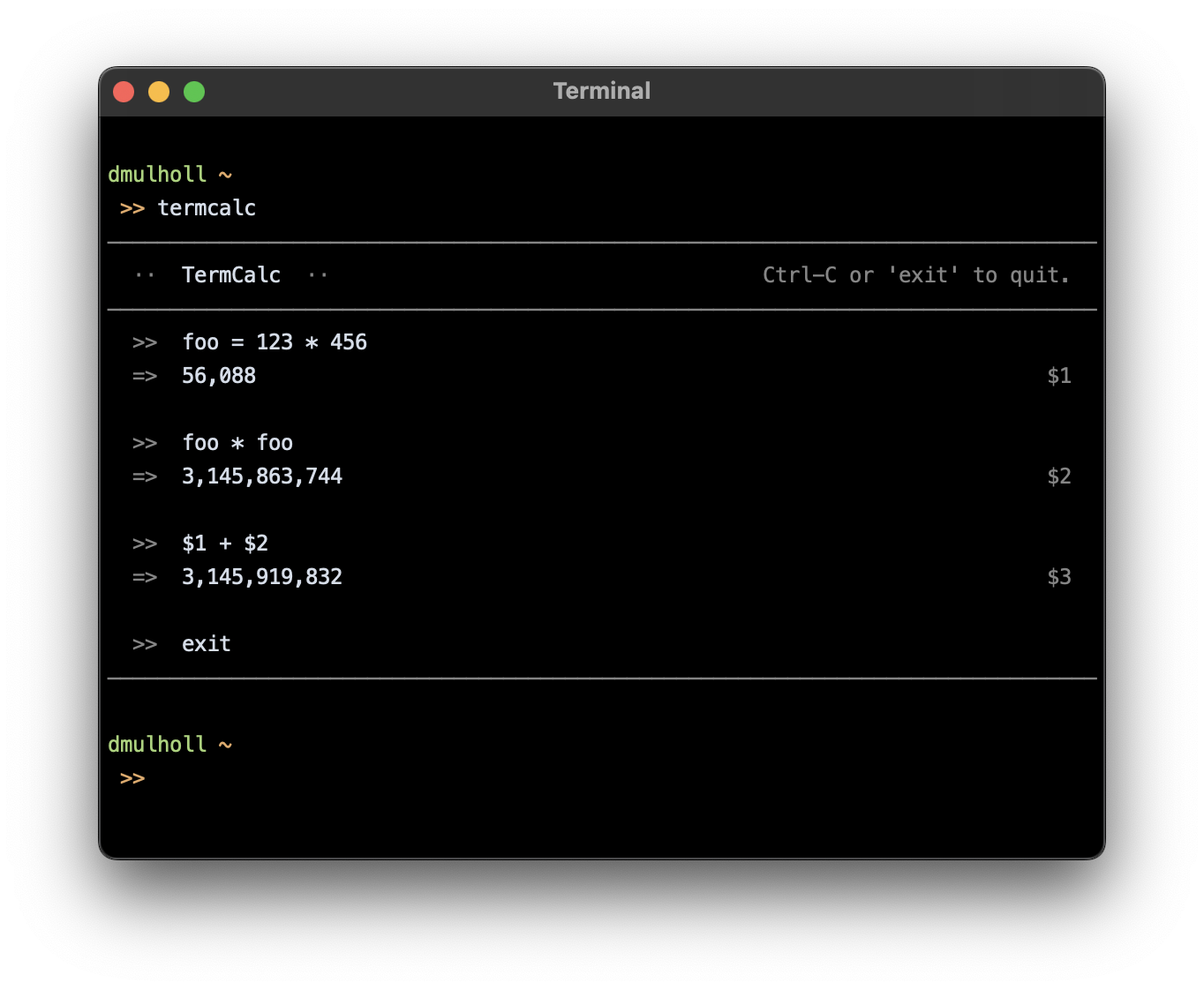# TermCalc

An interactive command line calculator.

TermCalc is a scientific calculator that runs in your terminal.TermCalc is written in Swift and is currently available only for macOS. The latest version is 1.3.0.

(Note that the binary isn't signed so macOS will make you jump through hoops before running it. Signed binaries are on the way.)

### Usage

Run `termcalc --help` to view the command line help:

```Usage: termcalc

TermCalc is an interactive command line calculator. All operations
are performed using IEEE 754 64-bit floats.

Options:
-d, --decimal <str>     Decimal separator (default '.').
-k, --kilo <str>        Thousands separator (default: ',').
-m, --milli <str>       Thousandths separator (default: ' ').
-p, --precision <int>   Decimal precision of output (default: 9).

Flags:
-h, --help              Print this help text and exit.
-v, --version           Print the version number and exit.
```

### Input

All mathematical operations are performed using IEEE 754 64-bit floats. Numbers can be entered in any of the following formats:

```123
123.456
0.123
.123
```

Exponential notation is also supported:

```1.23e4    ⟶   1.23 * 10 ^ 4    ⟶   12300
1.23e-4   ⟶   1.23 * 10 ^ -4   ⟶   0.000123
```

Binary, octal, decimal, and hexadecimal integer literals can be entered using a leading zero and letter prefix as below:

```0b101   ⟶   5
0o101   ⟶   65
0d101   ⟶   101
0x101   ⟶   257
```

You can use underscores to improve readability:

```pi = 3.141_592_654
```

### Operators

The following mathematical operators are supported:

```+    addition
-    subtraction
*    multiplication
/    division
%    remainder
^    power
!    factorial
```

Expressions can be nested in brackets to override the standard precedence rules, e.g `(3 + 4) * 5`.

### Variables

Variables are created by assigning to a name:

```foo = 2 + 3
```

Variable names can contain letters, underscores, and numbers, but must begin with either a letter or an underscore.

The following compound assignment operators are available for use with variables: `+=`, `-=`, `*=`, `/=`, `%=`, `^=`. The expression:

```foo += 1
```

is equivalent to

```foo = foo + 1
```

### Automatic Variables

The result of each evaluated expression is assigned to an automatic numbered variable: `\$1`, `\$2`, `\$3`, etc. (This name is displayed beside the result.)

The result of the last expression is always available via the automatic variable `\$`.

The mathematical constants `pi` and `e` are also available as preallocated variables.

### Functions

• Angles
```deg(x)        Convert x in radians to degrees.
```
• Trig
```cos(x)        Cosine of x; x in radians.
sin(x)        Sine of x; x in radians.
tan(x)        Tangent of x; x in radians.

cosd(x)       Cosine of x; x in degrees.
sind(x)       Sine of x; x in degrees.
tand(x)       Tangent of x; x in degrees.
```

Aliases with an explicit `r`-for-radians suffix are also available: `cosr`, `sinr`, `tanr`.

• Inverse Trig
```acos(x)       Inverse cosine of x; result in radians.
asin(x)       Inverse sine of x; result in radians.
atan(x)       Inverse tangent of x; result in radians.
atan(x,y)     Inverse tangent of y/x; result in radians,
sign determined by the quadrant of (x,y).

acosd(x)      Inverse cosine of x; result in degrees.
asind(x)      Inverse sine of x; result in degrees.
atand(x)      Inverse tangent of x; result in degrees.
atand(x,y)    Inverse tangent of y/x; result in degrees,
sign determined by the quadrant of (x,y).
```

Aliases with an explicit `r`-for-radians suffix are also available: `acosr`, `asinr`, `atanr`.

Longform (`arccos`) and shortform (`acos`) aliases are available for each function.

• Roots
```cbrt(x)       Cube root of x.
root(n,x)     Principal n-th root of x.
sqrt(x)       Square root of x.
```
• Logs
```ln(x)         Natural log of x.
log(b,x)      Base-b log of x.
log2(x)       Base-2 log of x.
log10(x)      Base-10 log of x.
```

### Keyboard Shortcuts

Standard line-editing keyboard shortcuts are supported:

```Ctrl-A    Move the cursor to the beginning of the line.
Ctrl-B    Move the cursor backwards.
Ctrl-C    Exit the application.
Ctrl-D    Delete the character at the cursor position.
Ctrl-E    Move the cursor to the end of the line.
Ctrl-F    Move the cursor forwards.
Ctrl-H    Delete the character to the left of the cursor.
Ctrl-K    Delete all characters to the right of the cursor.
Ctrl-L    Clear the screen.
Ctrl-U    Delete all characters to the left of the cursor.
Ctrl-W    Delete the previous word.
Ctrl-N    Scroll to the next history entry.
Ctrl-P    Scroll to the previous history entry.
```

The up and down arrow keys can also be used to scroll backwards and forwards through history entries.